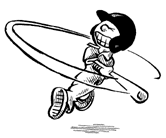Physics and Acoustics of Baseball & Softball Bats Daniel A. Russell, Ph.D. Graduate Program in Acoustics The Pennsylvania State University The contents of this page are ©2001-2011 Daniel A. Russell# Forces between Bat and Ball

 The impact between bat and ball is an extremely violent one, in which the bat imparts a huge force on the ball thereby causing it to change directions and gain speed. Consider a baseball weighing 5.125oz (mass = 0.145kg) which approaches the bat at a speed of 90mph (40.2m/s). After the collision with the bat, with a contact time of 0.7milliseconds (0.0007s)[1,2] the bat has a speed of 110mph (49.1m/s) in the opposite direction. Using Newton's second law we can estimate the average force acting on the ball during the hit: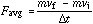Plugging in the numbers we find the average force to be Favg=18,436 N, which is equivalent to 4124 lbs of force. The impulse delivered by this force is the product of the average force the the contact time, resulting in an impulse of 12.91 Ns.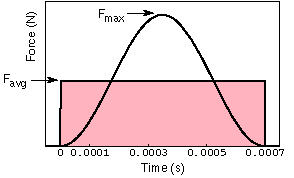The force that the bat exerts on the ball is not a constant during the entire duration of contact, but instead follows more of a sine-squared time history, starting and ending at zero and peaking approximately half way through the duration of contact. The figure at left illustrates this. The area under a force-vs-time curve is the implulse provided by the force. The average force, calculated above, is the constant force which acts for the same duration as the actual force, and encloses the same area under its force-vs-time curve (providing the same impulse) as does the actual force. Data for force-vs-time curves for a baseball may be reasonably well fit by a function of the form: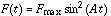where A=4500 to provide a 0.0007s contact time. The impulse (area under the curve) provided by this force may be obtained by integrating this force function over the contact time. We can estimate the peak force by setting this impulse equal to that provided by the average force, calculated above. Doing so results in a peak force of 36,982 N which is equivalent to 8314 lbs of force!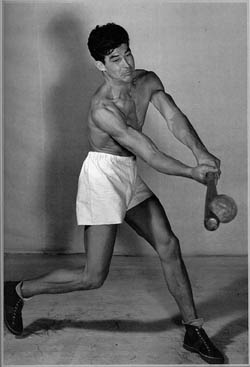The classic photograph taken by "Doc" Edgerton showing the moment of impact between bat and softball. The huge force exerted by the bat on the ball causes severe distortion of the ball as it is hit. Permission to use this image has been requested So, during the bat-ball collision, an average force of roughly two tons acts during the 0.7 millisecond contact time, with a peak force of about four tons. That's a lot of force! We can determine the average acceleration experienced by the ball from Newton's second law:  F=m a. Using the numbers above, we obtain an average acceleration of 127,145m/s2, or 12,740 times the acceleration due to gravity!

References
 Alan M. Nathan, "Dynamics of the baseball-bat collision," American Journal of Physics, 68(11), 979-990 (2000)
 Robert K. Adair, The Physics of Baseball, 3rd Edition, (Harper Collins, 2002)
 Rod, "The bounce of a ball," American Journal of Physics, 67(3), 222-227 (1999)

`  `

Back to Physics and Acoustics of Baseball & Softball Bats
`  `